# Bounded operator

Jump to: navigation, search

A mappingof a topological vector spaceinto a topological vector space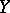such that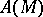is a bounded subset infor any bounded subsetof. Every operator, continuous on, is a bounded operator. If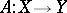is a linear operator, then forto be bounded it is sufficient that there exists a neighbourhoodsuch that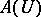is bounded in. Suppose thatandare normed linear spaces and that the linear operatoris bounded. ThenThis number is called the norm of the operatorand is denoted by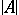. Then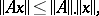andis the smallest constantsuch that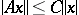for any. Conversely, if this inequality is satisfied, thenis bounded. For linear operators mapping a normed spaceinto a normed space, the concepts of boundedness and continuity are equivalent. This is not the case for arbitrary topological vector spacesand, but ifis bornological andis a locally convex space, then the boundedness of a linear operatorimplies its continuity. If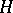is a Hilbert space and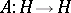is a bounded symmetric operator, then the quadratic form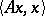is bounded on the unit ball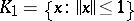. The numbersare called the upper and lower bounds of the operator. The pointsandbelongs to the spectrum of, and the whole spectrum lies in the interval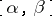. Examples of bounded operators are: the projection operator (projector) onto a complemented subspace of a Banach space, and an isometric operator acting on a Hilbert space.

If the spaceandhave the structure of a partially ordered set, for example are vector lattices (cf. Vector lattice), then a concept of order-boundedness of an operator can be introduced, besides the topological boundedness considered above. An operatoris called order-bounded ifis an order-bounded set infor any order-bounded setin. Examples: an isotone operator, i.e. an operator such thatimplies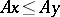.